# How to Add a Boolean Datatype Column to an Existing Table in SQL?

• Last Updated : 21 Nov, 2021

In SQL Server, a Boolean Datatype can be created by means of keeping BIT datatype. Though it is a numeric datatype, it can accept either 0 or 1 or NULL values only. Hence easily we can assign FALSE values to 0 and TRUE values to 1. This will provide the boolean nature for a data type.

Regarding the storage, if there are less than 9 columns of the bit data are present in the table,  they are stored as 1 byte. And for 9 to 16 BIT columns,  their consumption is 2 bytes and so on it will hold. In this article, let us how we can add a Boolean i.e. BIT datatype to an existing table in SQL SERVER.

Attention reader! Don’t stop learning now. Learn SQL for interviews using SQL Course  by GeeksforGeeks.

Let us assume there is a database “GEEKSFORGEEKS” is available and there is a table called “Authors” available with the following data.

Query:

```USE GEEKSFORGEEKS
SELECT * FROM Authors;```

Output: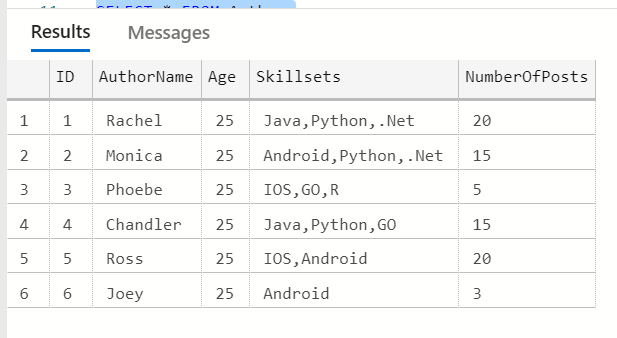Let us add a BIT data type to the “Authors” table. Bit datatype is helpful to represent Boolean nature either True(1) or False(0) and they are the only allowed values for BIT datatype.

```-- Add a column named "isActiveAuthor"
with BIT datatype for "Authors" table
ALTER TABLE Authors  ADD isActiveAuthor BIT;```

As there are already few rows available in the table, we can add a new column “isActiveAuthor” as a NULL pattern only. After that, we can update the data. After adding the column,

Query:

`SELECT * FROM Authors;`

Output: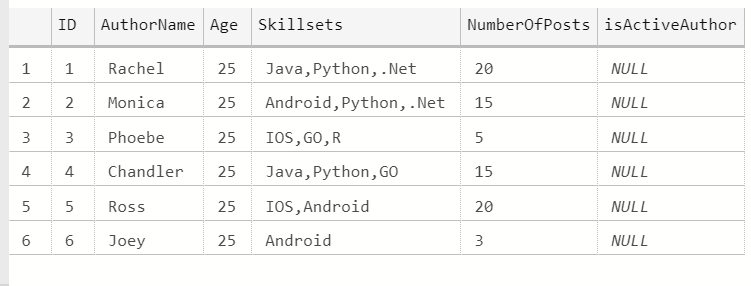So, when a BIT datatype column is added to an existing table, its value will be populated with “NULL” values.

Now let us try to update the column with a condition that if “NumberOfPosts” > 5, set “isActiveAuthor” to have the value 1 or else 0.

```-- Let us assume that if "NumberOfPosts"
is more than 5, author is active enough to write articles
-- Hence for this condition , set "isActiveAuthor"
column to have value 1
UPDATE Authors SET isActiveAuthor = 1 WHERE NumberOfPosts > 5;

-- On the other hand, if "NumberOfPosts"
is less  than and equal to 5,
-- author is not active in the recent days
and hence set "isActiveAuthor" column to have value 0
UPDATE Authors SET isActiveAuthor = 0 WHERE NumberOfPosts <= 5;

SELECT * FROM Authors;```

Output: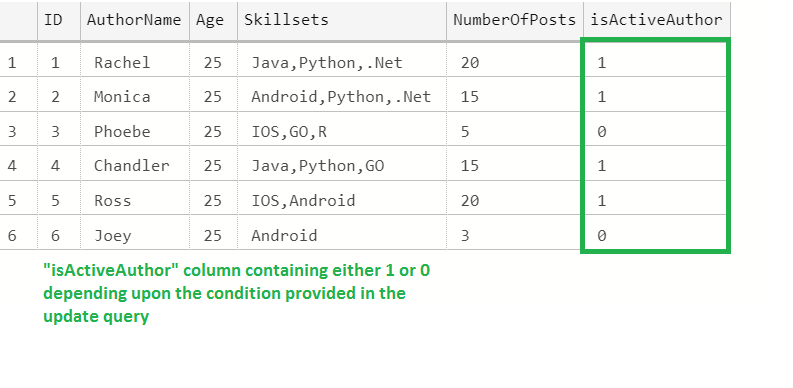As the BIT column supports only 0 or 1 as values, we have populated like above.

Let us try to update the value other than 0 or 1

```-- Trying to update the "isActiveAuthor"
with value 2, though errors are not
produced, output is different
UPDATE Authors SET isActiveAuthor =
2 WHERE NumberOfPosts = 5;

SELECT * FROM Authors;```

Output: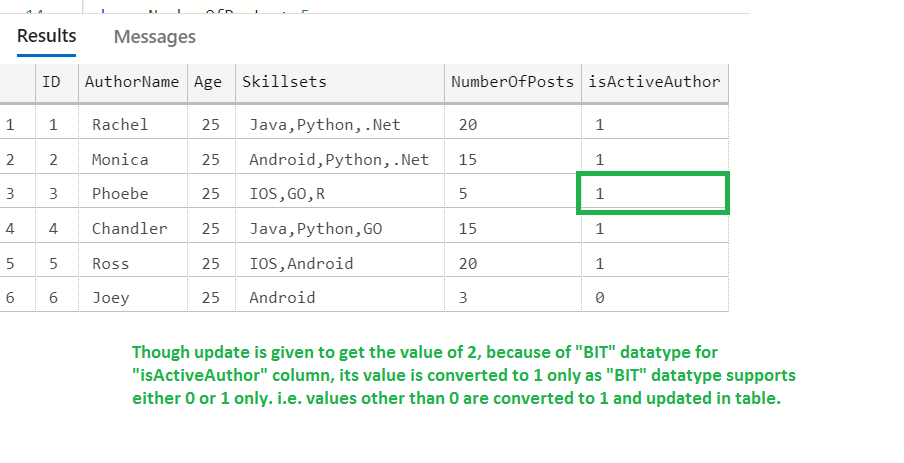Reason for the updated value of 1 in the 3rd row :

Though an update is given to get the value of 2, because of the “BIT” datatype for “isActiveAuthor” column, its value is converted to 1 only as the “BIT” datatype supports either 0 or 1 only. i.e. values other than 0 are converted to 1 and updated in the table.

We can check the same here with different examples

```DECLARE @isValid BIT

-- Initially it will have "NULL" value
SELECT @isValid AS BitType

--Assigning any nonzero value converts it to 1
-- i.e. other than 0, if any
value is provided , it is converted to 1
SET @isValid=9

SELECT @isValid AS BitType

--Assigning any nonzero value converts it to 1
SET @isValid=-100

SELECT @isValid AS BitType```

Output: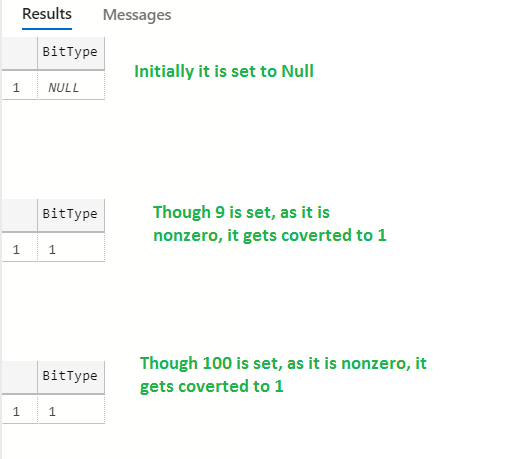This proves the “BIT” datatype accepts only the “Boolean” value i.e. either 0 or 1 only. If a nonzero value is given, it is converted to 1 only.